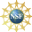# 8. Properties of Curves

## 2. Derivatives of Vector Functions

The definition of the derivative of a vector function mimics that for an ordinary function:

The derivative of a vector function $$\vec{f}(t)=\left\langle f_1(t),f_2(t),f_3(t)\right\rangle$$ is: $\dfrac{d\vec{f}}{dt}=\lim_{h\rightarrow 0}\dfrac{\vec{f}(t+h)-\vec{f}(t)}{h}$ provided the limit exists.

Although this definition is useful and essential for theoretical purposes, the following proposition give a formula which is much better for computational purposes.

The derivative of a vector function $$\vec{f}(t)=\left\langle f_1(t),f_2(t),f_3(t)\right\rangle$$ is: $\dfrac{d\vec{f}}{dt} =\left\langle \dfrac{df_1}{dt},\dfrac{df_2}{dt},\dfrac{df_3}{dt}\right\rangle$ provided the derivatives exists.

We use addition and scalar multiplication of vectors and the definition of a limit of a vector function: \begin{aligned} \dfrac{d\vec{f}}{dt} &=\lim_{h\rightarrow 0}\dfrac{\vec{f}(t+h)-\vec{f}(t)}{h} \\ &=\lim_{h\rightarrow 0} \dfrac{\left\langle f_1(t+h),f_2(t+h),f_3(t+h)\right\rangle -\left\langle f_1(t),f_2(t),f_3(t)\right\rangle}{h} \\ &=\lim_{h\rightarrow 0}\dfrac{\left\langle f_1(t+h)-f_1(t), f_2(t+h)-f_2(t),f_3(t+h)-f_3(t)\right\rangle}{h} \\ &=\lim_{h\rightarrow 0}\left\langle \dfrac{f_1(t+h)-f_1(t)}{h},\dfrac{f_2(t+h)-f_2(t)}{h}, \dfrac{f_3(t+h)-f_3(t)}{h}\right\rangle \\ &=\left\langle \lim_{h\rightarrow 0}\dfrac{f_1(t+h)-f_1(t)}{h}, \lim_{h\rightarrow 0}\dfrac{f_2(t+h)-f_2(t)}{h}, \lim_{h\rightarrow 0}\dfrac{f_3(t+h)-f_3(t)}{h}\right\rangle \\ &=\left\langle \dfrac{df_1}{dt},\dfrac{df_2}{dt},\dfrac{df_3}{dt}\right\rangle \end{aligned}

In other words, to take the derivative of a vector function we simply take the derivative of each component.

We can now define the velocity:

Given a parametric curve $$\vec{r}(t)=\left\langle x(t),y(t),z(t)\right\rangle$$, its velocity is its derivative: $\vec{v}(t)=\dfrac{d\vec{r}}{dt} =\left\langle \dfrac{dx}{dt},\dfrac{dy}{dt},\dfrac{dz}{dt}\right\rangle$

Find the velocity of the unit circle $$\vec{r}(t)=(\cos t,\sin t)$$. Plot the circle and several velocity vectors for $$0 \le t \le \dfrac{3\pi}{2}$$.

$$\vec{v}(t)=\dfrac{d\vec{r}}{dt}=\langle -\sin t,\cos t\rangle$$ \begin{aligned} &\vec{r}(0)=(1,0) &&\vec{v}(0)=\langle 0,1\rangle \\ &\vec{r}\left(\dfrac{\pi}{4}\right) =\left(\dfrac{1}{\sqrt{2}},\dfrac{1}{\sqrt{2}}\right) &&\vec{v}\left(\dfrac{\pi}{4}\right) =\left\langle \dfrac{-1}{\sqrt{2}},\dfrac{1}{\sqrt{2}}\right\rangle \\ &\vec{r}\left(\dfrac{\pi}{2}\right)=(0,1) &&\vec{v}\left(\dfrac{\pi}{2}\right)=\langle -1,0\rangle \\ &\vec{r}\left(\dfrac{3\pi}{4}\right) =\left(\dfrac{-1}{\sqrt{2}},\dfrac{1}{\sqrt{2}}\right)\qquad &&\vec{v}\left(\dfrac{3\pi}{4}\right) =\left\langle \dfrac{-1}{\sqrt{2}},\dfrac{-1}{\sqrt{2}}\right\rangle \\ &\vec{r}(\pi)=(-1,0) &&\vec{v}(\pi)=\langle 0,-1\rangle \\ &\vec{r}\left(\dfrac{5\pi}{4}\right) =\left(\dfrac{-1}{\sqrt{2}},\dfrac{-1}{\sqrt{2}}\right) &&\vec{v}\left(\dfrac{5\pi}{4}\right) =\left\langle \dfrac{1}{\sqrt{2}}, \dfrac{-1}{\sqrt{2}}\right\rangle \\ &\vec{r}\left(\dfrac{3\pi}{2}\right)=(0,-1) &&\vec{v}\left(\dfrac{3\pi}{2}\right) =\left\langle 1,0\right\rangle \\ \end{aligned}

In the above graph, notice that the velocity vectors are always tangent to the curve. This is no accident.

The velocity of a parametric curve is always tangent to the curve.

The proof depends heavily on the limit definition of the derivative. It is important to read the proof:

The velocity, $$\vec{v}$$, is the derivative of the position vector, $$\vec{r}$$. We write the derivative as a limit: $\vec{v}=\lim_{h\rightarrow 0}\dfrac{\vec{r}(t+h)-\vec{r}(t)}{h}$ The numerator is the
secant vector from $$\vec{r}(t)$$ to $$\vec{r}(t+h)$$.   (Shown in orange)
As $$h$$ gets smaller, the direction of the secant vector approaches the direction of the tangent vector, but (unfortunately) its length goes to zero. Dividing by $$h$$ gives the
secant quotient vector from $$\vec{r}(t)$$ to $$\vec{r}(t+h)$$.   (Shown in green)
Its direction is the same as that of the secant vector but its length may not go to zero. (Their lengths are equal when $$h=1$$.) If the limit exists, then the direction of $$\vec{v}$$ is the direction of the tangent vector.

The animation shows a parabola (in blue) along with
its tangent line at $$\vec{r}(2)$$ (in magenta)
its secant vectors at $$\vec{r}(2)$$ (in orange)
its secant quotient vectors at $$\vec{r}(2)$$ (in green).
As $$h$$ gets smaller, the directions of the secant vectors and the secant quotient vectors approach the direction of the tangent line. The lengths of the secant vectors approach $$0$$ but the lengths of the secant quotient vectors do not. For $$h \gt 1$$, the secant quotient is shorter than the secant, but for $$h \lt 1$$, the secant quotient is longer.

As a consequence of the proposition, we have:

For a parametric curve, the tangent vector is the velocity vector; they are synonyms.

Find the tangent vector to the curve $$\vec{r}(t)=\left(t,\dfrac{2}{t},t^{3}\right)$$.

$$\vec{v}(t)=\left\langle 1,\dfrac{-2}{t^2},3t^2\right\rangle$$

Just differentiate: $\vec{v}(t) =\dfrac{d\vec r}{dt} =\left\langle 1,\dfrac{-2}{t^2},3t^2\right\rangle$

Supported in part by NSF Grant #1123255